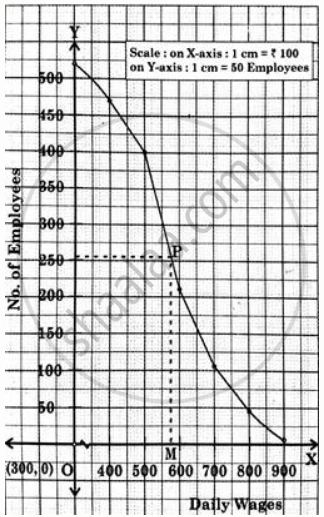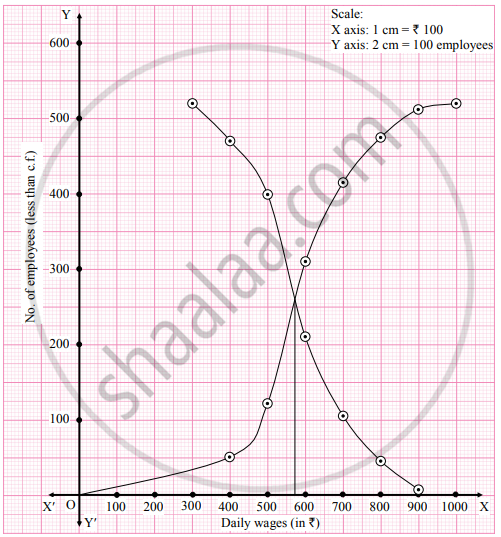# From the following distribution, determine median graphically. Daily Wages (in Rs.) No. of employees Above 300 520 Above 400 470 Above 500 399 Above 600 210 Above 700 105 Above 800 45 Above 900 7 - Mathematics and Statistics

Graph
Sum

From the following distribution, determine median graphically.

 Daily wages (in ₹) No. of employees Above 300 520 Above 400 470 Above 500 399 Above 600 210 Above 700 105 Above 800 45 Above 900 7

#### Solution 1

The given ‘more than cumulative frequency’ table is,

 Daily Wages (in Rs.) No. of employee (m.c.f) Above 300 520 Above 400 470 Above 500 399 Above 600 210 Above 700 105 Above 800 45 Above 900 7

The ‘more than ogive curve’ is plotted using the given lower limit of the class interval (x) against c.f. (y)
For median draw a line parallel to X-axis at Frequency "N"/(2) = (520)/(2) = 260#### Solution 2

To draw a ogive curve, we construct the less than and more than cumulative frequency table as given below:

 Daily wages (in ₹) No. of employees(f) Less than cumulative frequency(c.f.) More than cumulative frequency(c.f.) 300 − 400 50 50 520 400 − 500 71 121 470 500 − 600 189 310 399 600 − 700 105 415 210 700 − 800 60 475 105 800 − 900 38 513 45 900 − 1000 7 520 7 Total 520

The points to be plotted for less than ogive are (400, 50), (500, 121), (600, 310), (700, 415), (800, 475), (900, 513) and (1000, 520) and that for more than ogive are (300, 520), (400, 470), (500, 399), (600, 210), (700, 105), (800, 45), (900, 7).From the point of intersection of two ogives, we draw a perpendicular on X-axis.
The point where it meets the X-axis gives the value of the median.
∴ Median ≈ 574

Concept: Concept of Median
Is there an error in this question or solution?
Chapter 1: Partition Values - Exercise 1.3 [Page 19]

Share